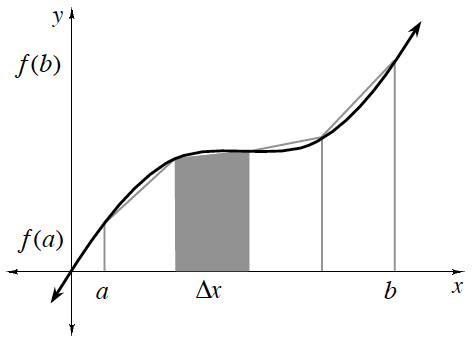### Home > APCALC > Chapter 2 > Lesson 2.1.2 > Problem2-28

2-28.

Write an equation that will approximate the area under $f (x) = \cos(x)$ over the interval $[ - \frac { \pi } { 2 } , \frac { \pi } { 2 } ]$ using six trapezoids with equal height. How can you use the fact that this is an even function to save yourself some work?

The Trapezoid RuleThe Trapezoid Rule can be used to approximate the area under a curve if the widths, $\Delta x$, of all trapezoids are of equal size:

$\left. \begin{array} { r } { A = \frac { \Delta x } { 2 } ( f ( a ) + 2 f ( a + \Delta x ) + 2 f ( a + 2 \Delta x ) + \ldots } \\ { + 2 f ( b - \Delta x ) + f ( b ) ) } \end{array} \right.$

Notice the symmetry about the $y$-axis. If we evaluate the trapezoidal sum on one side of the $y$-axis, can we determine the trapezoidal sum on the other side of the $y$-axis?